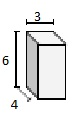# Volume of a Rectangular Prism Online Quiz

Following quiz provides Multiple Choice Questions (MCQs) related to Volume of a Rectangular Prism. You will have to read all the given answers and click over the correct answer. If you are not sure about the answer then you can check the answer using Show Answer button. You can use Next Quiz button to check new set of questions in the quiz.Q 1 - Find the volume of the following rectangular prism in cubic cm.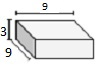### Explanation

Step 1:

Volume of rectangular prism = l × w × h cubic units

Step 2:

Volume of given prism = 9 × 3 × 9 = 243 cubic cm.

Q 2 - Find the volume of the following rectangular prism in cubic cm.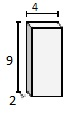### Explanation

Step 1:

Volume of rectangular prism = l × w × h cubic units

Step 2:

Volume of given prism = 4 × 9 × 2 = 72 cubic cm

Q 3 - Find the volume of the following rectangular prism in cubic cm.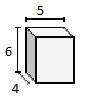### Explanation

Step 1:

Volume of rectangular prism = l × w × h cubic units

Step 2:

Volume of given prism = 5 × 6 × 4 = 120 cubic cm.

Q 4 - Find the volume of the following rectangular prism in cubic cm.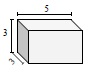### Explanation

Step 1:

Volume of rectangular prism = l × w × h cubic units

Step 2:

Volume of given prism = 5 × 3 × 3 = 45 cubic cm.

Q 5 - Find the volume of the following rectangular prism in cubic cm.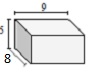### Explanation

Step 1:

Volume of rectangular prism = l × w × h cubic units

Step 2:

Volume of given prism = 9 × 5 × 8 = 360 cubic cm.

Q 6 - Find the volume of the following rectangular prism in cubic cm.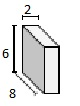### Explanation

Step 1:

Volume of rectangular prism = l × w × h cubic units

Step 2:

Volume of given prism = 2 × 6 × 8 = 96 cubic cm.

Q 7 - Find the volume of the following rectangular prism in cubic cm.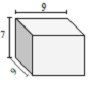### Explanation

Step 1:

Volume of rectangular prism = l × w × h cubic units

Step 2:

Volume of given prism = 9 × 7 × 9 = 567 cubic cm.

Q 8 - Find the volume of the following rectangular prism in cubic cm.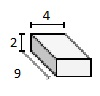### Explanation

Step 1:

Volume of rectangular prism = l × w × h cubic units

Step 2:

Volume of given prism = 4 × 2 × 9 = 72 cubic cm.

Q 9 - Find the volume of the following rectangular prism in cubic cm.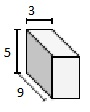### Explanation

Step 1:

Volume of rectangular prism = l × w × h cubic units

Step 2:

Volume of given prism = 3 × 5 × 9 = 135 cubic cm.

Q 10 - Find the volume of the following rectangular prism in cubic cm.